Courses

# Comparator - Digital Electronics, Electronics and Experimental Methods, CSIR-NET Physical Sciences Physics Notes | EduRev

## Physics for IIT JAM, UGC - NET, CSIR NET

Created by: Akhilesh Thakur

## Physics : Comparator - Digital Electronics, Electronics and Experimental Methods, CSIR-NET Physical Sciences Physics Notes | EduRev

The document Comparator - Digital Electronics, Electronics and Experimental Methods, CSIR-NET Physical Sciences Physics Notes | EduRev is a part of the Physics Course Physics for IIT JAM, UGC - NET, CSIR NET.
All you need of Physics at this link: Physics

The comparator is an electronic decision making circuit that makes use of an operational amplifiers very high gain in its open-loop state, that is, there is no feedback resistor.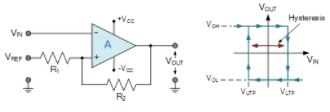The Op-amp comparator compares one analogue voltage level with another analogue voltage level, or some preset reference voltage, VREF and produces an output signal based on this voltage comparison. In other words, the op-amp voltage comparator compares the magnitudes of two voltage inputs and determines which is the largest of the two.

We have seen in previous tutorials that the operational amplifier can be used with negative feedback to control the magnitude of its output signal in the linear region performing a variety of different functions. We have also seen that the standard operational amplifier is characterised by its open-loop gain AO and that its output voltage is given by the expression: VOUT = AO(V+ – V-) where V+ and V- correspond to the voltages at the non-inverting and the inverting terminals respectively.

Voltage comparators on the other hand, either use positive feedback or no feedback at all (open-loop mode) to switch its output between two saturated states, because in the open-loop mode the amplifiers voltage gain is basically equal to AVO. Then due to this high open loop gain, the output from the comparator swings either fully to its positive supply rail, +Vcc or fully to its negative supply rail, -Vcc on the application of varying input signal which passes some preset threshold value.

The open-loop op-amp comparator is an analogue circuit that operates in its non-linear region as changes in the two analogue inputs, V+ and V- causes it to behave like a digital bistable device as triggering causes it to have two possible output states, +Vcc or -Vcc. Then we can say that the voltage comparator is essentially a 1-bit analogue to digital converter, as the input signal is analogue but the output behaves digitally.

Consider the basic op-amp voltage comparator circuit below.

Op-amp Comparator Circuit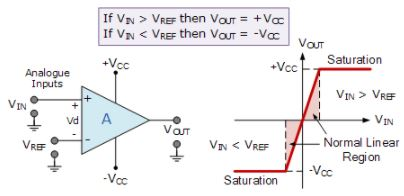With reference to the op-amp comparator circuit above, lets first assume that VIN is less than the DC voltage level at VREF, ( VIN < VREF ). As the non-inverting (positive) input of the comparator is less than the inverting (negative) input, the output will be LOW and at the negative supply voltage, -Vcc resulting in a negative saturation of the output.

If we now increase the input voltage, VIN so that its value is greater than the reference voltage VREF on the inverting input, the output voltage rapidly switches HIGH towards the positive supply voltage, +Vcc resulting in a positive saturation of the output. If we reduce again the input voltage VIN, so that it is slightly less than the reference voltage, the op-amp’s output switches back to its negative saturation voltage acting as a threshold detector.

Then we can see that the op-amp voltage comparator is a device whose output is dependant on the value of the input voltage, VIN with respect to some DC voltage level as the output is HIGH when the voltage on the non-inverting input is greater than the voltage on the inverting input, and LOW when the non-inverting input is less than the inverting input voltage. This condition is true regardless of whether the input signal is connected to the inverting or the non-inverting input of the comparator.

We can also see that the value of the output voltage is completely dependent on the op-amps power supply voltage. In theory due to the op-amps high open-loop gain the magnitude of its output voltage could be infinite in both directions, (±&#8734). However practically, and for obvious reasons it is limited by the op-amps supply rails giving VOUT = +Vcc or VOUT = -Vcc.

We said before that the basic op-amp comparator produces a positive or negative voltage output by comparing its input voltage against some preset DC reference voltage. Generally, a resistive voltage divider is used to set the input reference voltage of a comparator, but a battery source, zener diode or potentiometer for a variable reference voltage can all be used as shown.

Comparator Reference Voltages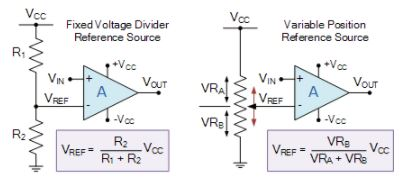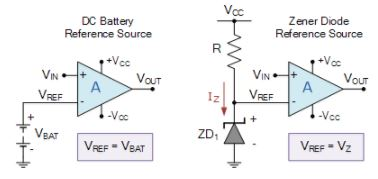In theory the comparators reference voltage can be set to be anywhere between 0v and the supply voltage but there are practical limitations on the actual voltage range depending on the op-amp comparator being device used.

Positive and Negative Voltage Comparators

A basic op-amp comparator circuit can be used to detect either a positive or a negative going input voltage depending upon which input of the operational amplifier we connect the fixed reference voltage source and the input voltage too. In the examples above we have used the inverting input to set the reference voltage with the input voltage connected to the non-inverting input.

But equally we could connect the inputs of the comparator the other way around inverting the output signal to that shown above. Then an op-amp comparator can be configured to operate in what is called an inverting or a non-inverting configuration.

Positive Voltage Comparator

The basic configuration for the positive voltage comparator, also known as a non-inverting comparator circuit detects when the input signal, VIN is ABOVE or more positive than the reference voltage, VREF producing an output at VOUT which is HIGH as shown.

Non-inverting Comparator Circuit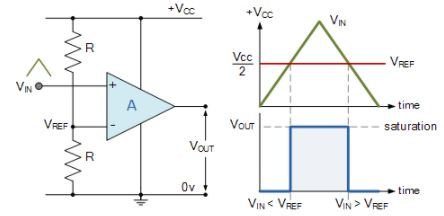In this non-inverting configuration, the reference voltage is connected to the inverting input of the operational amplifier with the input signal connected to the non-inverting input. To keep things simple, we have assumed that the two resistors forming the potential divider network are equal and: R1 = R2 = R. This will produce a fixed reference voltage which is one half that of the supply voltage, that is Vcc/2, while the input voltage is variable from zero to the supply voltage.

When VIN is greater than VREF, the op-amp comparators output will saturate towards the positive supply rail, Vcc. When VIN is less than VREF the op-amp comparators output will change state and saturate at the negative supply rail, 0v as shown.

Negative Voltage Comparator

The basic configuration for the negative voltage comparator, also known as an inverting comparator circuit detects when the input signal, VIN is BELOW or more negative than the reference voltage, VREF producing an output at VOUT which is HIGH as shown.

Inverting Comparator Circuit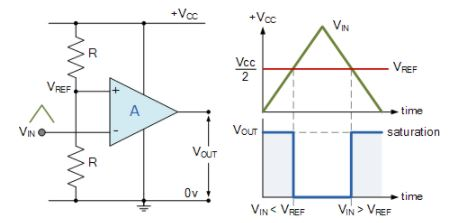In the inverting configuration, which is the opposite of the positive configuration above, the reference voltage is connected to the non-inverting input of the operational amplifier while the input signal is connected to the inverting input. Then when VIN is less than VREFthe op-amp comparators output will saturate towards the positive supply rail, Vcc.

Likewise the reverse is true, when VIN is greater than VREF, the op-amp comparators output will change state and saturate towards the negative supply rail, 0v.

Then depending upon which op-amp inputs we use for the signal and the reference voltage, we can produce an inverting or non-inverting output. We can take this idea of detecting either a negative or positive going signal one step further by combining the two op-amp comparator circuits above to produce a window comparator circuit.

Window Comparator

Window Comparator is basically the inverting and the non-inverting comparators above combined into a single comparator stage. The window comparator detects input voltage levels that are within a specific band or window of voltages, instead of indicating whether a voltage is greater or less than some preset or fixed voltage reference point.

This time, instead of having just one reference voltage value, a window comparator will have two reference voltages implemented by a pair of voltage comparators. One which triggers an op-amp comparator on detection of some upper voltage threshold, VREF(UPPER)and one which triggers an op-amp comparator on detection of a lower voltage threshold level, VREF(LOWER). Then the voltage levels between these two upper and lower reference voltages is called the “window”, hence its name.

Using our idea above of a voltage divider network, if we now use three equal value resistors so that R1 = R2 = R3 = R we can create a very simple window comparator circuit as shown. Also as the resistive values are all equal, the voltage drops across each resistor will also be equal at one-third the supply voltage, 1/3Vcc. Then in this simple example, we can set the upper reference voltage to 2/3Vcc and the lower reference voltage to 1/3Vcc. Consider the window comparator circuit below.

Window Comparator Circuit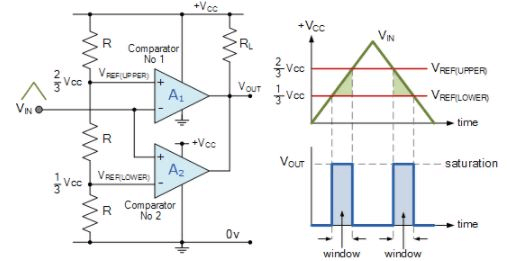When VIN is below the lower voltage level, VREF(LOWER) which equates to 1/3Vcc, the output will be LOW. When VIN exceeds this 1/3Vcc lower voltage level, the first op-amp comparator detects this and switches the output HIGH to Vcc.

As VIN continues to increase it passes the upper voltage level, VREF(UPPER) at 2/3Vcc and the second op-amp comparator detects this and switches the output back LOW. Then the difference between VREF(UPPER) and VREF(LOWER) (which is 2/3Vccc – 1/3Vcc in this example) creates the switching window for the positive going signal.

Lets now assume that VIN is at its maximum value and equal to Vcc. As VIN decreases it passes the upper voltage level VREF(UPPER) of the second op-amp comparator which switches the output HIGH. As VIN continues to decrease it passes the lower voltage level, VREF(LOWER) of the first op-amp comparator once again switching the output LOW.

Then the difference between VREF(UPPER) and VREF(LOWER) creates the window for the negative going signal. So we can see that as VIN passes above or passes below the upper and lower reference levels set by the two op-amp comparators, the output signal VOUTwill be HIGH or LOW.

In this simple example we have set the upper trip level at 2/3Vcc and the lower trip level at 1/3Vcc (because we used three equal value resistors), but can be any values we choose by adjusting the input thresholds. As a result, the window width can be customized for a given application.

If we used a dual power supply and set the upper and lower trip levels to say ±10 volts and VIN was a sinusoidal waveform, then we could use this window comparator circuit as a zero crossing detector of the sine wave which would produce an output, HIGH or LOW every time the sine wave crossed the zero volts line from positive to negative or negative to positive.

We can take this idea of detecting voltage levels further by connecting a number of different op-amp comparators together with them all using a common input signal, but with each comparator using a different reference voltage set by our now familiar voltage divider network across the supply. Consider the voltage level detector circuit below.

Comparator Voltage Level Detector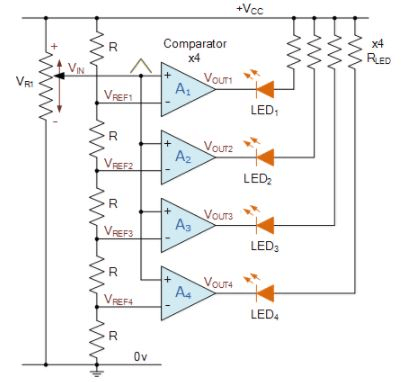As above, the voltage divider network provides a set of reference voltages for the individual op-amp comparator circuits. To produce the four reference voltages will require five resistors. The junction at the bottom pair of resistors will produce a reference voltage that is one-fifth the supply voltage, 1/5Vcc using equal value resistors. The second pair 2/5Vcc, a third pair 3/5Vcc and so on, with these reference voltages increasing by a fixed amount of one-fifth (1/5) towards 5/5Vcc which is actually Vcc.

As the common input voltage increases, the output of each op-amp comparator circuit switches in turn starting with the lower comparator, A4 and upwards towards A1 as the input voltage increases. So by setting the values of the resistors in the voltage divider network, the comparators can be configured to detect any voltage level. One good example of the use of voltage level detection and indication would be for a battery condition monitor.

Also by increasing the number of op-amp comparators in the set, more trigger points can be created. So for example, if we had eight op-amp comparators in the chain and fed the output of each comparator to an 8-to-3 line Digital Encoder, we could make a very simple analogue-to-digital converter, (ADC) that would convert the analogue input signal into a 3-bit binary code (0-to-7).

Op-amp Comparator with Positive Feedback

We have seen here that operational amplifiers can be configured to operate as comparators in their open-loop mode, and this is fine if the input signal varies rapidly or is not too noisy. However if the input signal, VIN is slow to change or electrical noise is present, then the op-amp comparator may oscillate switching its output back and forth between the two saturation states, +Vcc and -Vcc as the input signal hovers around the reference voltage, VREF level. One way to overcome this problem and to avoid the op-amp from oscillating is to provide positive feedback around the comparator.

As its name implies, positive feedback is a technique for feeding back a part or fraction of the output signal that is in phase to the non-inverting input of the op-amp via a potential divider set up by two resistors with the amount of feedback being proportional to their ratio.

The use of positive feedback around an op-amp comparator means that once the output is triggered into saturation at either level, there must be a significant change to the input signal VIN before the output switches back to the original saturation point. This difference between the two switching points is called hysteresis producing what is commonly called a Schmitt trigger circuit. Consider the inverting comparator circuit below.

Inverting Op-amp Comparator with Hysteresis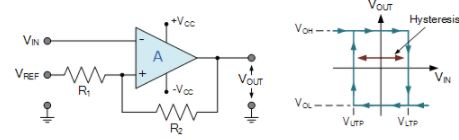For the inverting comparator circuit above, VIN is applied to the inverting input of the op-amp. Resistors R1 and R2 form a voltage divider network across the comparator providing the positive feedback with part of the output voltage appearing at the non-inverting input. The amount of feedback is determined by the resistive ratio of the two resistors used and which is given as:

Voltage Divider Equation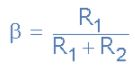Where: β (beta) can be used to indicate the feedback fraction.

When the input signal is less than the reference voltage, VIN < VREF, the output voltage will be HIGH, VOH and equal to the positive saturation voltage. As the output is HIGH and positive, the value of the reference voltage on the non-inverting input will be approximately equal to: +β*Vcc called the Upper Trip Point or UTP.

As the input signal, VIN increases it becomes equal too this upper trip point voltage, VUTPlevel at the non-inverting input. This causes the comparators output to change state becoming LOW, VOL and equal to the negative saturation voltage as before.

But the difference this time is that a second trip point voltage value is created because a negative voltage now appears at the non-inverting input which is equal to: -β*Vcc as a result of the negative saturation voltage at the output. Then the input signal must now fall below this second voltage level, called the Lower Trip Point or LTP for the voltage comparators output to change or switch back to its original positive state.

So we can see that when the output changes state, the reference voltage at the non-inverting input also changes creating two different reference voltage values and two different switching points. One called the Lower Upper Trip Point (UTP) and the other being called the Lower Trip Point (LTP). The difference between these two trip points is called Hysteresis.

The amount of hysteresis is determined by the feedback fraction, β of the output voltage fed back to the non-inverting input. The advantage of positive feedback is that the resulting comparator Schmitt trigger circuit is immune to erratic triggering caused by noise or slowly changing input signals within the hysteresis band producing a cleaner output signal as the op-amp comparators output is only triggered once.

So for positive output voltages, VREF = +β*Vcc, but for negative output voltages, VREF = -β*Vcc. Then we can say that the amount of voltage hysteresis will be given as: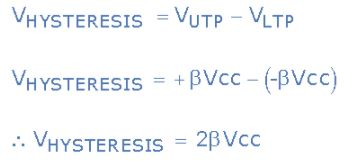We can also produce a non-inverting op-amp comparator circuit with built in hysteresis by changing the input and reference terminals as shown:

Non-inverting Op-amp Comparator with Hysteresis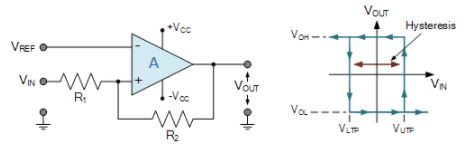Note that the arrows on the hysteresis graph indicate the direction of switching at the upper and lower trip points.

Comparator Example No1

An operational amplifier is to be used with positive feedback to produce a Schmitt trigger circuit. If resistor, R1 = 10kΩ and resistor, R2 = 90kΩ, what will be the values of the upper and lower switching points of the reference voltage and the width of the hysteresis if the op-amp is connected to a dual ±10v power supply.

Given: R1 = 10kΩ, R2 = 90kΩ. Power supply +Vcc = 10v and -Vcc = 10v.

Feedback Fraction: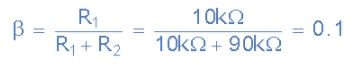Upper Voltage Trip Point, VUTP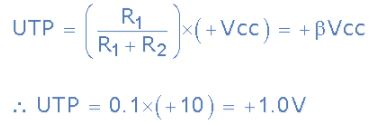Lower Voltage Trip Point, VLTP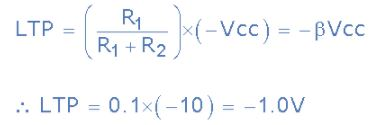Hysteresis width: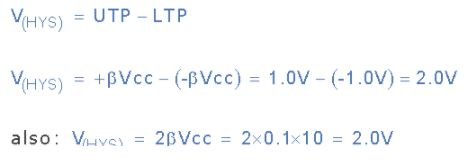Then the reference voltage VREF, switches between +1V and -1V as the output saturates from one level to the other. Hopefully we can see from this simple example that the width of this hysteresis, 2 volts in total, can be made larger or smaller simply by adjusting the voltage divider ratio of the feedback resistors R1 and R2.

The Voltage Comparator

Although we can use operational amplifiers such as the 741 as a basic comparator circuit, the problem with this is that op-amps are only optimised for linear operation. That is where the input terminals are at virtually the same voltage level and its output stage is designed to produce a linear output voltage that is not saturated for long periods of time. Also standard operational amplifiers are designed to be used in closed-loop applications with negative feedback from its output to its inverting input.

A dedicated voltage comparator on the other hand is a non-linear device that allows for heavy saturation, due to its very high gain, when the input signals differs by a relatively small amount. The difference between an op-amp comparator and a voltage comparator is in the output stage as a standard op-amp has an output stage that is optimized for linear operation, while the output stage of a voltage comparator is optimized for continuous saturated operation as it is always intended to be close to one supply rail or the other and not in between.

Commercial comparators such as the LM311 single comparator, the LM339 quad comparator or the LM393 dual differential comparator, are voltage comparators which come in a standard IC package operating from a single or dual supply. These dedicated voltage comparators are designed for the sole purpose of switching the output very quickly from one saturated state the another as the transistors used for a voltage comparators output stage are generally switching transistors.

Since voltage comparators convert a linear input signal into a digital output signal, they are commonly used to connect two dissimilar electrical signals with different supply or reference voltages. As a result the output stage of the voltage comparator is generally configured as a single open collector (or Drain) transistor switch with open or closed states rather than actual output voltages as shown.

Voltage Comparator Circuit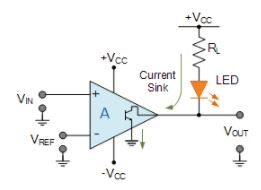Here, the open collector output from the voltage comparator is connected to a voltage source via a single pull-up resistor (and an LED for indication) which pulls the single output high to the power supply. When the output switch is HIGH it creates a high impedance path, therefore no current flows as VOUT = Vcc.

When the comparator changes state and the output switch is LOW, it creates a low impedance path to ground and current flows through the pull-up resistor (and LED) causing a voltage drop across itself with the output being pulled to the lower supply level, ground in this case.

Then we can see that there is very little difference between the schematic symbol of an op-amp comparator and a voltage comparator or their internal circuits. The main difference is in the output stage with the open collector or drain configuration is useful for driving relays, lamps, etc. By driving a transistor from the output allows for a greater switching current capacity than that of the comparators output alone.

Op-amp Comparator Summary

In this tutorial about the Op-amp Comparator we have seen that a comparator circuit is basically an operational amplifier without feedback, that is, the op-amp is used in its open-loop configuration, and when the input voltage, VIN exceeds a preset reference voltage, VREF, the output changes state.

Due to the very high open-loop gain of the operational amplifier, using it with positive feedback or even with no feedback at all causes the output to saturate to its supply rail producing one of two distinct output voltages depending on the relative values of its two inputs. This bistable behaviour is non-linear and forms the basis of op-amp comparator and Schmitt trigger circuits.

The output stages of dedicated comparators, such as the single LM311, the dual LM393 or the quad LM339 are designed to operate in their saturation regions allowing these voltage comparator circuits to be widely used in analogue-to-digital converter applications and for various types of voltage level detection circuits.

The erratic switching behaviour of an open-loop comparator can be easily overcome by adding positive feedback between the output and input of the comparator. With positive feedback, the circuit has hysteresis with the output switching occurring between two different switching points, UTP and LTP.

Op-amp window comparators are a type of voltage comparator circuit which uses two op-amp comparators to produce a two-state output that indicates whether or not the input voltage is within a particular range or window of values by using two reference voltages. An upper reference voltage and a lower reference voltage.

While operational amplifiers and comparators may look similar, they are very different and designed to be used in different applications as an op-amp may be used as a comparator, a voltage comparator can not be used as an op-amp due to its non-linear output stage.

We know from previous tutorials that an operational amplifier is an analogue device with a differential analogue input and an analogue output and if operated in its open-loop configuration its output acts like a comparator output. But dedicated voltage comparators (LM311, LM393, LM339) are widely available which will perform much better than a standard op-amp comparator.

Offer running on EduRev: Apply code STAYHOME200 to get INR 200 off on our premium plan EduRev Infinity!

159 docs

,

,

,

,

,

,

,

,

,

,

,

,

,

,

,

,

,

,

,

,

,

,

,

,

,

,

,

;